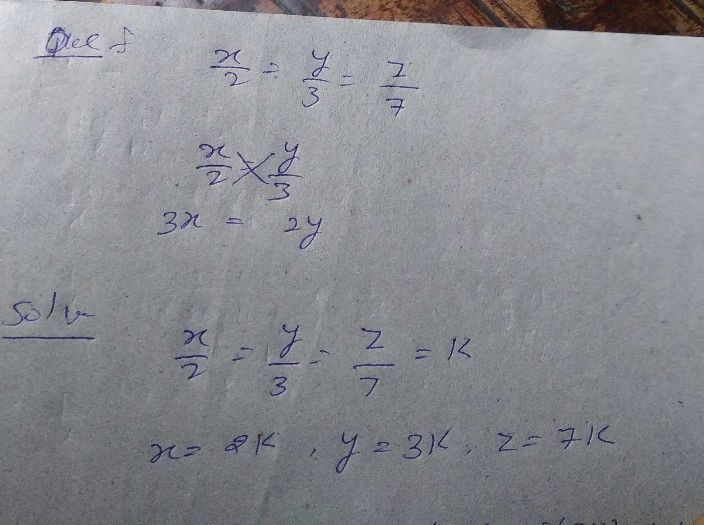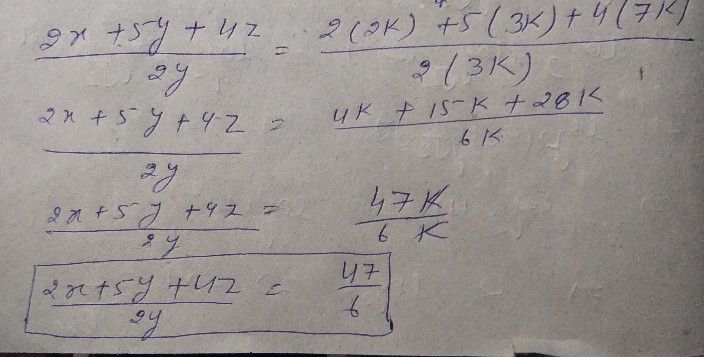Symbol
Problem$14$ aft $x/2=y/3=z/7,\pi$ at $-$ $\left(2x-5y+4z\right)/2y\pi$ (a) $6/23$ $\left(b\right)2316$ $\left(c\right)3/2$ $15$ $x$ $y=2$ $.3$ $y$ $x=4$ $.1.2$ $\bar{\pi 0}$
Other
SolutionQanda teacher - Laxmithere is a problem in the options. again check the answerStudent
wrong
17/6